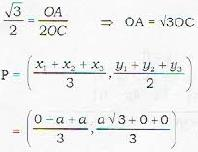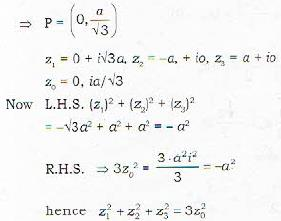Courses

# Test:- Complex Number - 2

## 20 Questions MCQ Test Topic-wise Tests & Solved Examples for IIT JAM Mathematics | Test:- Complex Number - 2

Description
This mock test of Test:- Complex Number - 2 for Mathematics helps you for every Mathematics entrance exam. This contains 20 Multiple Choice Questions for Mathematics Test:- Complex Number - 2 (mcq) to study with solutions a complete question bank. The solved questions answers in this Test:- Complex Number - 2 quiz give you a good mix of easy questions and tough questions. Mathematics students definitely take this Test:- Complex Number - 2 exercise for a better result in the exam. You can find other Test:- Complex Number - 2 extra questions, long questions & short questions for Mathematics on EduRev as well by searching above.
QUESTION: 1

### The equation represents | z + 3 | - | z - 5 | = 0

Solution: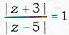, it is bisector of line segment joining (-3.,0) and (5,0)

QUESTION: 2

### Point z with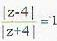in the complex plane lies on

Solution: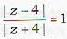I z - 4 I = | z + 4|
If ( z - 4 ) = k |z + 4| k ≠ 1
(x - y)2 + y2 = ± (x+y)2 + y2 then it is circle
but now x = 0
x = 0 hence it is y - axis.

QUESTION: 3

### The locus of z satisfying the condition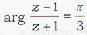is

Solution: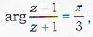it is a circle

QUESTION: 4

The inequality | z - 4 | < | z - 2 | region given by

Solution:

| z - 4 | < | z - 2 |
=> | x - 4 + iy | < | x + 2 - iy |
=> (x - y)2 + y2 < (x - 2)2 + y2
=> x2- 8 x + 16 < x- 4 x +4
=> - 4 x < -12
=> x > 3
=> real value of z should be > 3

QUESTION: 5

Which of the following is correct

Solution:

The concept of inequality does not hold in the set of complex number

QUESTION: 6

If z = x - iy and w = (1 - iz)/( z - l ) , then | w | = 1 implies that in the complex plane, z iies on

Solution:

Let. z = x + iy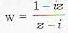and |w| = 1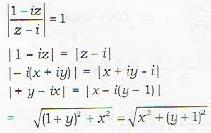squaring both side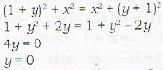Hence z = x + iy = x + 0
which is real

QUESTION: 7

If the complex number z1, z2, z3 are in A.P. then they lies on a

Solution:

z1, z2, z3, are in AP, so they lie on a straight line (by mid point method)

QUESTION: 8

The value of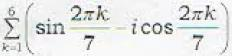is

Solution: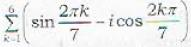is
= -i (sum of complex roots of 1)
= i

QUESTION: 9

The point z, z2, z3, z4 in the complex plane are the vertices of a parallelogram taken in order if and only if.

Solution:

z1, z2, z3, z4, are the complex numbers which are the vertices of a parallelogram taken in order if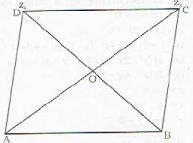Diagonal of a parelielogam bisect each other at midpoint of AC and BD
∴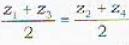z1 + z3 = z+ z4

QUESTION: 10

If (1, ω, ω2)5 are the cube root of unity, then (1 - ω + ω2)5 + (1 + ω - ω2)5 =

Solution:

1, ω, ω2 are cube roots of one (unity).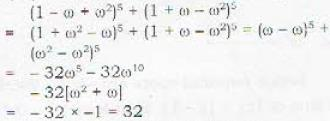QUESTION: 11

If a, b are the complex cube roots of unity and x = a + b, y = ωa + bω2, z = aω2 - bω, then xyz =

Solution:

If a and b are cube roots of unity
x = a + b , y = ωa + bω2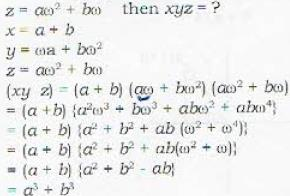QUESTION: 12

If 1, ω, ω2 ... ωn-1 are the nth roots of unity then (1 - ω) (1 - ω2) ... (1 — ωn-2) is equal to

Solution:

If 1, ω, ω2..., ωn-1 are the nth root of unity then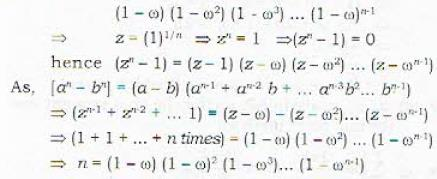QUESTION: 13

If ω, ω2 are the complex root of unity, then [(a + bω - cω2)/(c + aω + bω2)] + [(a + bω+ aω2)/ (b + cω + aω2)]

Solution:

if ω1, ω2, are the complex roots of unity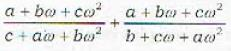= (ω2) + (ω)

QUESTION: 14

If cube root of unity are 1, ω, ω2, then the roots of the equation (x - 1)3 + 8 = 0 are

Solution:

1 ,ω, ωare root of cube root of unity , then
(x - 1)3 + 8 = 0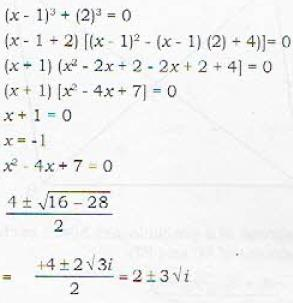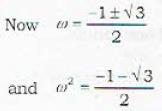hence required roots are - 1 , 1 - 2w , 1 - 2w

QUESTION: 15

For any complex number z, the minimum value of |z| + | z - 1 | is

Solution:

Value of |z| + |z - 1| is at least |1 - 0 |
so minimum value of |z| + |z - 1| is 1

QUESTION: 16

Multiplying a complex number z by 1 + i rotates the radius vector of z by an angle of

Solution:

tan θ = 1/1
θ = π/4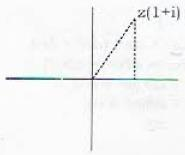QUESTION: 17

If |z+ z2| = |z1| + |z2| , then arg z1 - arg zis equal to

Solution: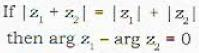QUESTION: 18

The area of the triangle formed by the complex number z, iz, z + iz in the argand plane is

Solution: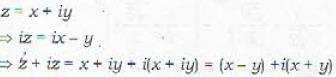Area, AABC =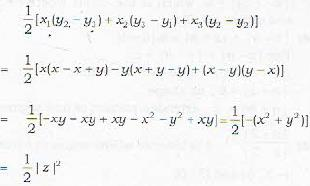QUESTION: 19

Let z be a complex number. Then the angle between z and iz is

Solution:

As eπ/2 is i so, iz is obtained by rotating z by an angle of π/2. so , angle between z and iz is π/2.

QUESTION: 20

If z1, z2, z3 are vertices of equilateral triangle with zo as centroid, then z12 + z22 + z32 is equal to

Solution:

A, B, C are equilateral A and P be the centroid of ΔABC.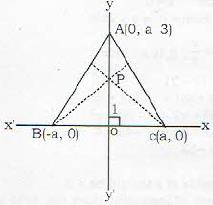since AB = BC = CA = 2a
Δ AOC
sin 60 = AO/CA